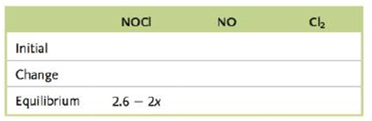# In a given experiment, 5.2 moles of pure NOCl was placed in an otherwise empty 2.0-L container. Equilibrium was established by the following reaction: 2 NOCl ( g ) ⇌ 2 NO ( g ) + Cl 2 ( g ) K = 1.6 × 10 − 5 a . Using numerical values for the concentrations in the Initial row and expressions containing the variable x in both the Change and Equilibrium rows, complete the following table summarizing what happens as this reaction reaches equilibrium. Let x = the concentration of Cl 2 that is present at equilibrium. b. Calculate the equilibrium concentrations for all species.### Chemistry: An Atoms First Approach

2nd Edition
Steven S. Zumdahl + 1 other
Publisher: Cengage Learning
ISBN: 9781305079243

#### Solutions

Chapter
Section### Chemistry: An Atoms First Approach

2nd Edition
Steven S. Zumdahl + 1 other
Publisher: Cengage Learning
ISBN: 9781305079243
Chapter 12, Problem 95CWP
Textbook Problem
28 views

## In a given experiment, 5.2 moles of pure NOCl was placed in an otherwise empty 2.0-L container. Equilibrium was established by the following reaction: 2 NOCl ( g ) ⇌ 2 NO ( g ) + Cl 2 ( g )       K = 1.6 × 10 − 5 a. Using numerical values for the concentrations in the Initial row and expressions containing the variable x in both the Change and Equilibrium rows, complete the following table summarizing what happens as this reaction reaches equilibrium. Let x = the concentration of Cl2 that is present at equilibrium.b. Calculate the equilibrium concentrations for all species.

(a)

Interpretation Introduction

Interpretation: The ICE-table (Initial, change and equilibrium concentration) corresponds to the given reaction is to be completed. The equilibrium concentrations of all the species are to be calculated.

Concept introduction: The equilibrium constant K describes the ratio of the reactant to the product on the equilibrium conditions in terms of molar concentration.

The equilibrium constant depends upon temperature.

Law of mass action is applicable on the equilibrium reactions.

The concentration unit mol/L is represents as M .

To determine: The values of concentrations of all the species at the initial, change and equilibrium positions.

### Explanation of Solution

Explanation

Given

The reaction is given as,

2NOCl(g)2NO(g)+Cl2(g)

The initial moles of NOCl(g) is 5.2mol .

The volume of the container is 2.0L .

The equilibrium constant is 1.6×105 .

The concentration of Cl2 at equilibrium is x .

The initial concentration of NOCl(g) is calculated by the formula,

C=nV

Where,

• C is the concentration.
• n is the number of moles.
• V is the volume.

Substitute the values of n and V in the above formula.

C=nV=5.2mol2L=2.6mol/L=2.6M_

The ICE-table in terms of concentration for the reaction is given as,

2NOCl(g)2NO(g)+Cl2(g)Initial2.6M00Change2x+2x+xEquilibrium2.6-x2xx

The equilibrium constant in terms of concentration for the above reaction is calculated by the formula,

K=[NO]2[Cl2][NOCl]2

Where,

• K is the equilibrium constant.
• [NO] is the equilibrium concentration of NO(g) .
• [Cl2] is the equilibrium concentration of Cl2(g) .
• [NOCl] is the equilibrium concentration of NOCl(g)

(b)

Interpretation Introduction

To determine: The equilibrium concentrations of all the species.

### Still sussing out bartleby?

Check out a sample textbook solution.

See a sample solution

#### The Solution to Your Study Problems

Bartleby provides explanations to thousands of textbook problems written by our experts, many with advanced degrees!

Get Started

Find more solutions based on key concepts
The smallest unit of any substance is the _____. a. atom b. molecule c. cell

Biology: The Unity and Diversity of Life (MindTap Course List)

Which of Earths internal zones is the largest?

Fundamentals of Physical Geography

B vitamins often act as: a. antioxidants. b. blood clotting factors. c. coenzymes. d. none of the above.

Nutrition: Concepts and Controversies - Standalone book (MindTap Course List)

Give general chemical formulas for a primary, a secondary, and a tertiary amine.

Chemistry for Today: General, Organic, and Biochemistry

Even a small loss of body weight can improve OA.

Nutrition Through The Life Cycle

What evidence can you give that our Galaxy has a galactic corona?

Foundations of Astronomy (MindTap Course List)

How are zooplankton different from phytoplankton? Heterotroph from autotroph?

Oceanography: An Invitation To Marine Science, Loose-leaf Versin

One end of a cord is fixed and a small 0.500-kg object is attached to the other end, where it swings in a secti...

Physics for Scientists and Engineers, Technology Update (No access codes included)